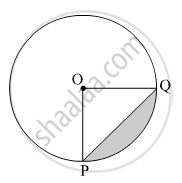SSC (Marathi Semi-English) 10thMaharashtra State Board
Share

# A Chord Pq of a Circle with Radius 15 Cm Subtends an Angle of 60° with the Centre of the Circle. Find the Area of the Minor as Well as the Major Segment. ( π = 3.14, √ 3 = 1.73) - SSC (Marathi Semi-English) 10th - Geometry

ConceptAreas of Sector and Segment of a Circle

#### Question

A chord PQ of a circle with radius 15 cm subtends an angle of 60° with the centre of the circle. Find the area of the minor as well as the major segment. ( $\pi$ = 3.14,  $\sqrt{3}$ = 1.73)

#### Solution

Radius of the circle, r = 15 cm
Let O be the centre and PQ be the chord of the circle.∠POQ = θ = 60º
Area of the minor segment = Area of the shaded region

$= r^2 \left( \frac{\pi\theta}{360°} - \frac{\sin\theta}{2} \right)$
$= \left( 15 \right)^2 \times \left( \frac{3 . 14 \times 60° }{360° } - \frac{\sin60° }{2} \right)$
$= 225 \times \frac{3 . 14}{6} - 225 \times \frac{\sqrt{3}}{4}$
$= 117 . 75 - 97 . 31$
$= 20 . 44 {cm}^2$

Now,
Area of the circle =

$\pi r^2 = 3 . 14 \times \left( 15 \right)^2 = 3 . 14 \times 225$  = 706.5 cm2
∴ Area of the major segment = Area of the circle − Area of the minor segment = 706.5 − 20.44 = 686.06 cm2
Thus, the areas of the minor segment and major segment are 20.44 cm2 and 686.06 cm2, respectively.

Is there an error in this question or solution?

#### APPEARS IN

Solution A Chord Pq of a Circle with Radius 15 Cm Subtends an Angle of 60° with the Centre of the Circle. Find the Area of the Minor as Well as the Major Segment. ( π = 3.14, √ 3 = 1.73) Concept: Areas of Sector and Segment of a Circle.
S